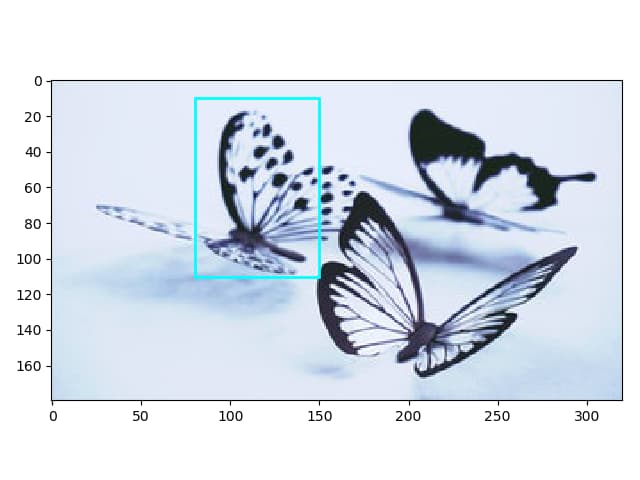# Draw Rectangle on Image in Matplotlib

Jinku Hu Apr 12, 2021 Mar 01, 2020 Matplotlib Matplotlib Patch

When we need to draw a rectangle on an image or plain figure in Matplotlib, the `rectangle` patch from `matplotlib.patches` should be added to the axes by `add_patch` method.

A Matpotlib `patch` is a 2-D artist that has a face and edge color. Matplotlib has `patches` like,

• `Arc`
• `Arrow`
• `ArrowStyle`
• `BoxStyle`
• `Circle`
• `CirclePolygon`
• `ConnectionPatch`
• `ConnectionStyle`
• `Ellipse`
• `FancyArrow`
• `FancyArrowPatch`
• `FancyBboxPatch`
• `Patch`
• `Path`
• `PathPatch`
• `Polygon`
• `Rectangle`
• `RegularPolygon`
• `Shadow`
• `Wedge`

`Rectangle` patch draws a rectangle with a specified coordinate of lower left corner and specified width and height.

``````Rectangle(xy, width, height, angle=0.0, fill=True, **kwargs)
``````

`xy` is a length-2 tuple to specify the coordinates of lower left corner of the rectangle to be drawn,

`width` is the rectangle’s width,

`height` is the rectangle’s height,

`angle` is the anti-clockwise angle in degrees.

`fill` specifies whether the rectangle is filled with the `facecolor`.

Some valid `kwargs` are,

• `edgecolor`: the color of edges of the rectangle
• `facecolor`: the filled color of the rectangle

## Draw Rectangle in Matplotlib

``````import matplotlib.pyplot as plt
import matplotlib.patches as patches

fig, ax = plt.subplots()

ax.plot([1,4],[1,4])

patches.Rectangle(
(1, 1),
0.5,
0.5,
edgecolor = 'blue',
facecolor = 'red',
fill=True
) )

plt.show()
``````

The rectangle patch is created by the constructor,

``````patches.Rectangle(
(1, 1),
1,
2,
edgecolor = 'blue',
facecolor = 'red',
fill=True
)
``````

Its left bottom corner’s coordinate is `(1, 1)`, its width is `1` and height is `2`.

The edge color is specified as `blue`, meanwhile, the filled color is `red`.

`add_patch(P)` method of `Axes` object adds a `Patch` - `p` to the `axis`.If `fill` is set to be `False`, the rectangle will not be filled even `facecolor` is specified with some color.

``````import matplotlib.pyplot as plt
import matplotlib.patches as patches

fig, ax = plt.subplots()

ax.plot([1,4],[1,4])

patches.Rectangle(
(1, 1),
1,
2,
edgecolor = 'blue',
facecolor = 'red',
fill=False
) )

plt.show()
``````## Draw Rectangle on Image in Matplotlib

If we need to draw a rectangle on an image in Matplotlib, it is similar to the above approach but with the extra step to get the `Axes` handle.

``````import matplotlib.pyplot as plt
import matplotlib.patches as patches
from PIL import Image

im = Image.open('butterfly.jpg')

plt.imshow(im)

ax = plt.gca()

rect = patches.Rectangle((80,10),
70,
100,
linewidth=2,
edgecolor='cyan',
fill = False)

`plt.imshow(im)` displays the image `butterfly.jpg` and the handle of `Axes` could be got by the function `plt.gca()` whose `gca` means `get current axes`.